# New Simplified Chemistry Class 9 ICSE Solutions Study of The First Element Hydrogen

## New Simplified Chemistry Class 9 ICSE Solutions Study of The First Element Hydrogen

Viraf J Dalal Chemistry Class 9 Solutions and Answers

Equation Worksheet

Complete and balance the equations
Hydrogen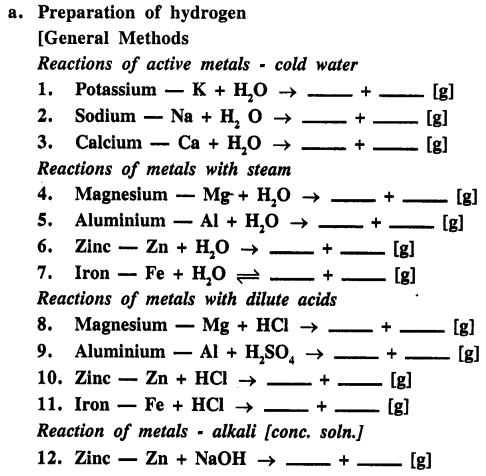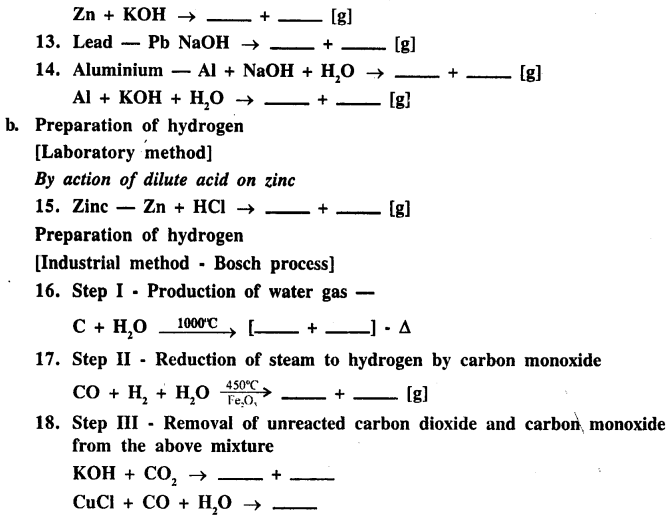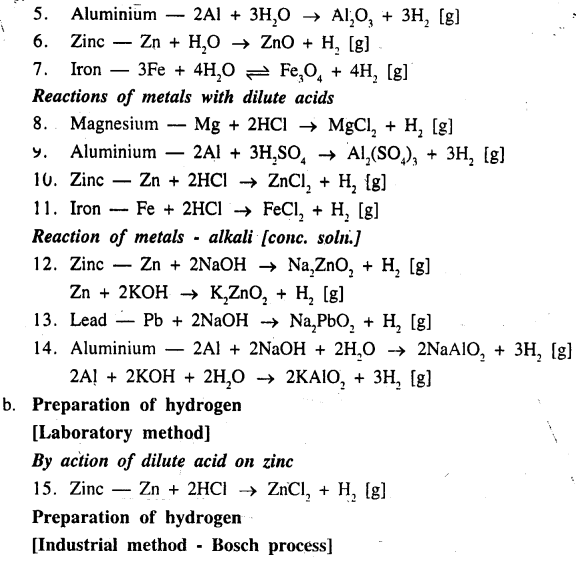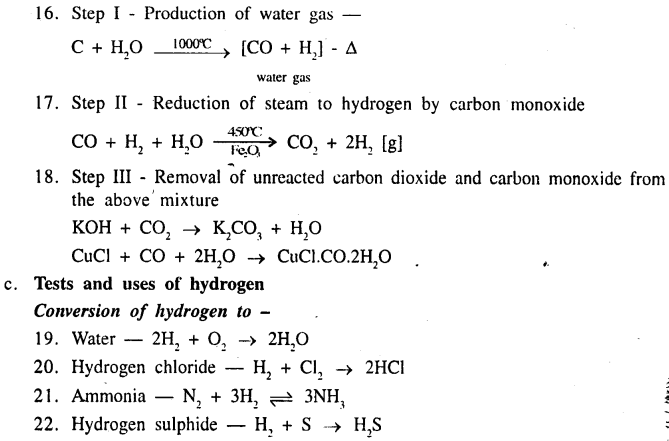Exercise

Question 1.(1984)
Name an element whsich reacts violently with water at room temperature.
Element reacts with water violently at room temperature is potassium.

Question 2.(1984)
What do the following symbols [or formula] denote : 2H ; H2 ; H+. [two atoms, molecule, ion]
2H donotes — 2 atoms of hydrogen
H2 — a molecule of hydrogen
H+ — an ion

Question 3.(1984)
Write correctly balanced equation for the following “word equation” :
calcium + water → calcium hydroxide + hydrogen
Ca + 2H2O → Ca[OH]2 + H2

Question 4.(1984)
When steam is passed over red-hot iron, magnetic oxide of iron and hydrogen are obtained. “The reaction between steam and red-hot iron is a Reversible Reaction.” What is meant by this statement.
Statement means magnetic oxide of iron and hydrogen recombine to form iron and steam.

Question 5.(1984)
How can you obtain hydrogen from sodium hydroxide [not by electrolysis].
When powdered zinc reacts with sodium hydroxide it produces hydrogen.Question 1.(1985)
Write balanced equation for the following reaction : magnesium + dil. hydrochloric acid →
Mg + 2HCl (dil.) → MgCl2 + H2

Question 1.(1986)
Name a gas which burns in air or oxygen forming water.
The gas that burns in oxygen to form water is hydrogen.

Question 2.(1986)
Write correctly balanced equation for the following : When steam is passed over red hot iron.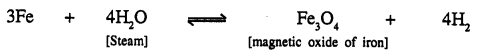Question 3.(1986)
Explain the following : Two jars of H2 are collected – “one burns quietly and the other does not”.
‘One bums quietly’ is pure hydrogen and ‘which does not bum quietly’ is mixed hydrogen with air it explodes on burning.

Question 1.(1987)
Write correctly the balanced equation for the following : ‘When zinc filings are added to a concentrated solution of sodium hydroxide’.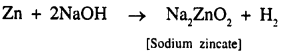Question 2.(1987)
Describe one chemical test applied to the following gases, which would enable you to distinguish between them :‘carbon monoxide and hydrogen’.Question 1.(1988)
Write down the “word equation” for the following reaction : sodium hydroxide solution + zinc →
‘Word equation’
Sodium hydroxide + zinc → Sodium zincate + hydrogen

Question 2.(1988)
Explain briefly how hydrogen is manufactured on a large scale, from steam.
Manufacture of H2 from steam :
From natural gas : Natural gas is obtained from petroleum wells and consists mainly CH4 (methane). It is mixed with steam at 30 atm. and passed over heated nickel 800°C, when water gas is formed.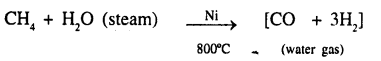Separation of CO : The gaseous mixture of [CO and H2] is passed through ammonical cuprous chloride solution in order to dissolve uncombined CO.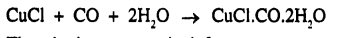Thus hydrogen gas is left over.

Question 1.(1989)
State the products of the reaction “when steam is passed over red-hot iron”.When steam is passed over red hot iron, teriferric tetroxide and hydrogen are the ; products formed.

Question 1.(1990)
How can you obtain hydrogen from a mixture of hydrogen and carbon monoxide.
See Q. 2. 1988. By dissolving the mixture in ammonical cuprous chloride. CO dissolves and H2 is left over.

Question 2.(1990)
What do you observe when a piece of sodium is dropped into cold water ?
When a piece of sodium metal dropped in cold water we observe : Sodium floats on water surface melts forming a silvery globule which darts about the surface of water catches fire and burns with golden yellow flame.
Bubbles of hydrogen evolve and solution is soapy, slightly warm (alkaline) hence turns red litmus blue.

Question 3.(1990)
Give reasons for the following : ‘Though hydrogen is lighter than air, it is not collected by the downward displacement of air’.
Though hydrogen is lighter than air it is not collected by displacement of air as it forms explosive mixture with air.

Question 4.(1990)
Complete the following word equations :

1. Sodium hydroxide + zinc → hydrogen + ____
2. Calcium + water → calcium hydroxide + ____

1. Sodium hydroxide + zinc → hydrogen + sodium zincate
2. Calcium + water → calcium hydroxide + hydrogen

Question 1.(1991)
How would you obtain ‘hydrogen from sodium hydroxide’ solution other than by electrolysis ?
By adding aluminium powder in cone, sodium hydroxide.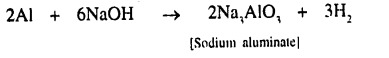Question 1.(1992)
Complete and balance the following equations :
Al + NaOH + ____ → ____ + ____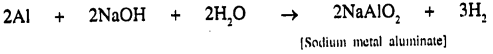Question 2.(1992)
What do the following symbols represent : 2H and H2.
2H → represents → 2 atoms of hydrogen
H2 → represents → 1 molecule of hydrogen

Question 1.(1993)
Write balanced equation of the reaction in the preparation of : hydrogen from a solution of potassium hydroxide [other than by electrolysis].
Preparation of H2 from potassium hydroxide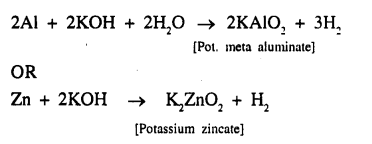Question 2.(1993)
Describe briefly, with equations, the Bosch Process for the large scale production of hydrogen.
Bosch process for large production of H2 :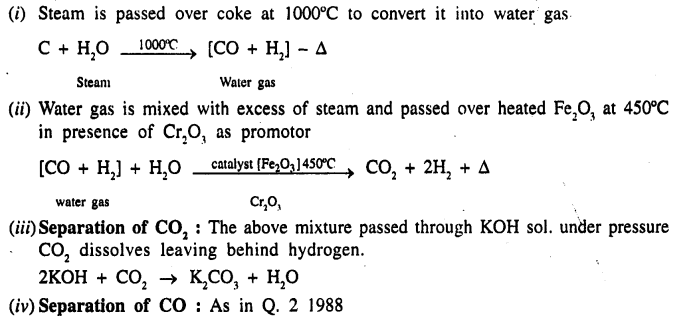Question 3.(1993)
Account for the following facts :

1. Though lead is above hydrogen in the activity series, it does not react with dilute hydrochloric acid or dilute sulphuric acid.
2. Potassium and sodium are not used to react with dilute ‘hydrochloric acid or dilute sulphuric acid in the laboratory preparation of hydrogen.

1. Pb (lead) is above hydrogen in reactivity series. With dil. HCl, Pb forms PbCl2 and with dil. H2SO4, Pb forms PbSO4 both PbCl2 and PbSO4 are insoluble and forms respective coating to stop further reaction.
2. Sodium and potassium with dil. HCl or dil. H2SO4 but reaction is highly explosive and practically not-feasible.

Question 1.(1994)
Place the metals calcium, iron, magnesium and sodium in order of their activity with water, placing the most active first. Write the equation for each of the above metals which react with Water.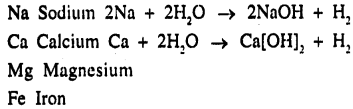Question 2.(1994)
Why is copper not used to prepare hydrogen by the action of dilute hydrochloric acid or dilute sulphuric acid on the metal, [copper [Cu] below hydrogen – no reaction]
Cu is below hydrogen in reactivity series and cannot displace H2 from acid and no-reaction takes place.

Question 1.
State the electronic configuration of hydrogen [at. no. 1].e
Give a reason why hydrogen can be placed in group 1 [1A] and group 17 [VIIA] of the periodic table.
Electronic configuration of hydrogen is 1 i.e. it has le, IP in nucleus. Hydrogen can be placed in group 1[1A] as it forms a positive ion as in HC1 like alkali metals H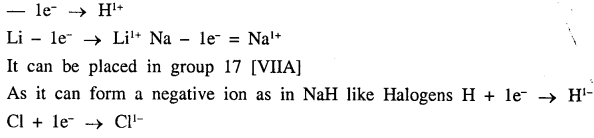Question 2.
Give the general group characteristics applied to hydrogen with respect to similarity in properties of hydrogen with –

(a) alkali metals of group 1 [IA]
(b) halogens of group 17 [VIIA].

with special reference to valency electrons & ion formation.
General group characteristics of
(a) Alkali metals of group 1 [IA] : Alkali metals lose electron to become electro positive ion
Na – le → Na1+
H – le → H1+
(b) Hydrogen gains 1 electron like halogens to be come electronegative ion
Cl + le → Cl1-
H + le → H1-

Question 3.
How did the name ‘hydrogen’ originate. How does hydrogen occur in the combined state.
Hydrogen means in Greek – water former. Hydrogen initially called inflammable gass that it bums in air. It produces water. Lavoisier in 1783 established its name ‘hydrogen’ meaning water producer hydrogen in combined state : in a animal and plant tissues. As a constituent of : proteins, carbohydrates, fats, acids, alkalis, petrolium products and organic substances.

Question 4.
Give balanced equation for obtaining hydrogen from cold water using –

(a) A monovalent active metal
(b) A divalent active metal

(a) Monovalent active metal Na :
2Na + 2H2O → 2NaOH + H2
(b) Divalent active metal Ca :
Ca + 2H2O → Ca[OH]2 + H2

Question 5.
Give balanced equations for obtaining hydrogen from ?

(a) Boiling water using a divalent metal
(b) Steam using a trivalent metal
(c) Steam using a metal – and the reaction is reversible.

To obtain H2 using boiling water and
(a) A divalent metal Mg
Mg + H2O → MgO + H2
(b) Steam and trivalent metal Al
2Al + 3H2O → Al2O3 + 3H2
(c) Steam and a metal – a reaction is reversible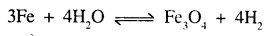Question 6.
State why hydrogen is not prepared in the laboratory by the action of –

(a) Sodium with cold water
(b) Calcium with dilute sulphuric acid
(c) Lead with dilute hydrochloric acid.

(a) As sodium is very reactive and reacts with water violently, darts on the surface of water and collection of hydrogen becomes difficult.
(b) The reaction between calcium and dil. H2SO is highly explosive and practically not feasible.
(c) With lead dil. HCl forms PbCl2 which is in soluble and forms coating and stops the further reaction.

Question 7.
Give balanced equations for the following conversions.

(a) Sodium zincate from zinc
(c) Sodium aluminate from aluminium.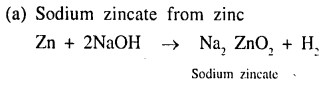Question 8.
In the laboratory preparation of hydrogen from zinc and dil. acid. Give reasons for the following :

(a) The complete apparatus is air-tight.
(b) Dilute nitric acid is not preferred as the reactant acid.
(c) The lower end of the thistle funnel should dip below the level of the acid in the flask.
(d) Hydrogen is not collected over air.

(a) The gas is highly inflammable, any leakage can cause explosion.
(b) Hydrogen produced is oxidised to water as nitric acid is powerful oxidizing agent.
(c) Otherwise gas produced will escape through thistle funnel.
(d) Air forms an explosive mixture with H2.

Question 9.
‘Magnesium reacts with very dilute nitric acid at low temperatures liberating hydrogen.’ Give reasons.
Magnesium reacts with dil. HNO3 at low temperature liberating H2, since oxidising action of dil. HNO3 is much reduced due to dilution.

Question 10.
State the conditions and give balanced equations for the conversion of – (a) coke to water gas, (b) water gas to hydrogen – in the Bosch process.
Conditions and balance equations for conversion of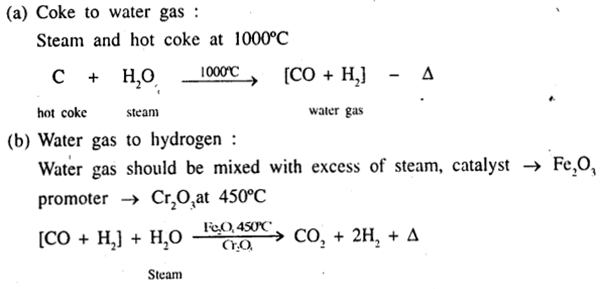Question 11.
How are the unreacted gases separated out in ‘Bosch process’ in the manufacture of hydrogen.
See Q. 2 Step (iii) and (iv) 1993
CO2 is removed by dissolving mixture in water under pressure or in caustic potash solution to dissolve CO2. CO is removed by dissolving mixture in ammonical CuCl solution. CuCl + CO + 2H2O → CuCl. C0.2H2O.

Question 12.
Compare the combustibility of –

(a) pure hydrogen
(b) hydrogen-air mixture.

Combustibility of :
(a) Pure hydrogen : Bums quietly in air with a pale blue flame forming water
2H2 + O2 → 2H2O
(b) Hydrogen air mixture : It explodes on burning.

Question 13.
State the reactant added to hydrogen to obtain the respective product in each case.

(a) Ammonia
(b) Hydrogen chloride
(c) Water
(d) Hydrogen sulphide

(a) Ammonia : Nitrogen and hydrogen.
(b) Hydrogen chloride : Hydrogen and chlorine.
(c) Water : Hydrogen and oxygen.
(d) Hydrogen sulphide : Hydrogen and sulphur.

Question 14.
State the use of hydrogen –

(a) As a fuel
(b) In hydrogenation of oil & coal
(c) In extraction of metals

Use of hydrogen :
(a) As a fuel in the form of coal gas, water gas, liquid hydrogen.
(b) In hydrogenation of oil & coal :

1. Vegetable oil [palm oil] turns to [vegetable ghee] semisolid fats by hydrogenation in presence of nickel catalyst at 170°C.
2. Passage of H2 under high pressure in presence of catalyst and at suitable temperature produces a product similar to petroleum.

(c) In extraction of metals : by reducing oxides of metals.

Question 15.
Explain the terms – oxidation and reduction in terms of addition and removal of oxygen/hydrogen with suitable examples.
OXIDATION : “Addition of oxygen or removal of hydrogen is called oxidation.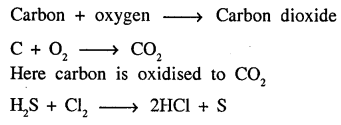Here H2S is oxidised to sulphur as removal of H2 takes place.
Reduction : Addition of hydrogen or removal of oxygen is called reduction.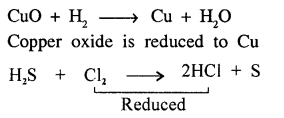chlorine is reduced, as addition of hydrogen.

Question 16.
Explain the term – redox reaction with an example involving – the reaction of hydrogen sulphide with chlorine.
Redox Reaction : “The reaction in which oxidation and reduction takes place simultaneously is called Redox Reaction.”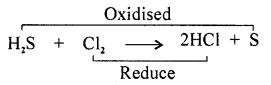Removal of hydrogen is oxidation. Here H2S is oxidised to sulphur whereas addition of hydrogen is reduction.
Here chlorine is being reduced to hydrogen chloride

Question 17.
State what are – oxidising and reducing agents. Give examples of oxidising and reducing agents in the gaseous, liquid and solid form. Give two tests each generally answered by oxidising and reducing agents respectively.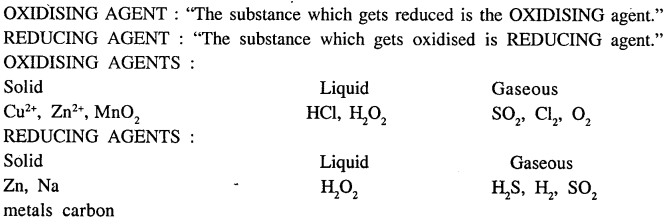Test For An Oxidising Agent :

1. On heating strongly, oxidising agent liberates oxygen and to test oxygen : O2 gas rekindles a glowing splinter.
2. On warming oxidising agent with cone. HCl, it liberates chlorine, that bleaches moist litmus paper.

Test For A Reducing Agent :

1. When reducing agent is warmed with HNO3, BROWN FUMES of N02 are given out.
2. Reducing agent, decolourises the pink colour of (KMnO4) dil. potassium permanganate solution.

Hydrogen – Unit Test Paper 6

Q.1. Select from A to G the reactant added, to give the products 1 to 5, in the preparation of hydrogen gas.
A : dilute acid
B : dilute alkali
C : cold water
D : cone, alkali
E : boiling water
F : cone, acid
G : steam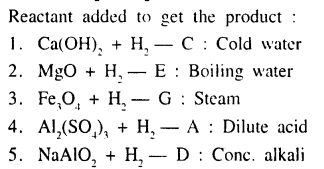Q.2. Give balanced equations for the following conversions, 1 to 5.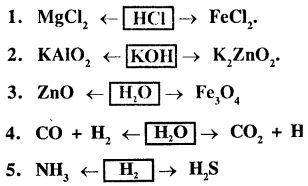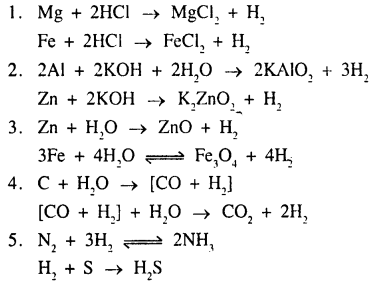Q.3. Give reasons for the following.

1. Nitric acid in the dilute form is not used in the laboratory preparation of hydrogen from metals.
2. Granulated zinc is preferred to metallic zinc in the preparation of hydrogen using dilute acid.
3. Hydrogen and alkali metals of group 1 [IA] react with copper [II] oxide to give copper.
4. Hydrogen is collected by the downward displacement of water and not air even though – it is lighter than air.
5. A mixture of hydrogen and chlorine can be separated by passage through a porous pot.

1. Nitric acid is a strong oxidising agent and nascent oxygen formed oxidises hydrogen produced to water.
2. Zinc granules are preferred rather than pure zinc as impurity copper present in it has a catalysing effect and speeds up the rate of the reaction.
3. Hydrogen and alkali metals act as reducing agent and reduce CuO to Cu.
CuO + H2 → Cu + H2O
4. H2 is insoluble in water. Moreover H, forms explosive mixture with air.
5. H2 and Cl2 differ in densities H2 = 0.9 g/lit
Cl2 = 35.5 g/lit
H2 diffuses faster than Cl2 and is separated.

Q.4. Name the following.

1. A metal below iron but above copper in the activity series of metals which has no reaction with water.
2. A metal which cannot be used for the preparation of hydrogen using dilute acids.
3. The salt formed when aluminium reacts with potassium hydroxide, during the preparation of hydrogen from alkalis.
4. A gaseous reducing agent which is basic in nature.
5. A compound formed between hydrogen and an element from group 17 [VIIA] – period 3.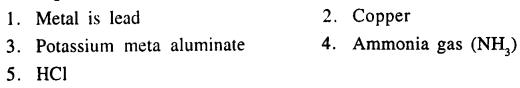Q.5. Select the correct answer from the symbols in bracket.

1. The element placed below hydrogen in group 1 [IA]. [Na, Li, K, F],
2. The element other than hydrogen, which forms a molecule containing a single covalent bond. [Cl, N, O]
3. The element, which like hydrogen has one valence electron. [He, Na, F, O]
4. The element, which like hydrogen is a strong reducing agent. [Pb, Na, S, Cl]
5. The element which forms a diatomic molecule. [C, Br, S, P]

1. Li
2. Cl
3. Na
4. Na
5. Br

Q.6. The diagram represents the preparation & collection of hydrogen by a standard laboratory method.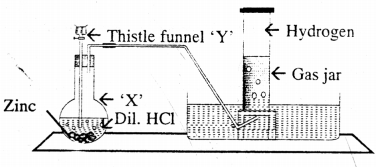1. State what is added through the thistle funnel ‘Y’
2. State what difference will be seen if pure zinc is added in the distillation flask ‘X’ instead of granulated zinc.
3. Name a solution which absorbs the impurity – H2S.
4. State why hydrogen is collected after all the air in the apparatus is allowed to escape.
5. Name a gas other than hydrogen collected by the same method.